# The Ultimate pH Primer - Are you Familiar with Hydrogen?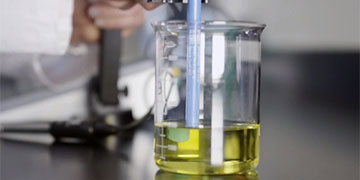So, we've all heard of pH but how many of you know what pH stands for?  Go ahead, take a guess before reading on. The term pH is derived from a combination of 'p' for the word power and 'H' representing the element of Hydrogen. Together, pH means 'the power of hydrogen'. The measurement of pH is a convenient way for us to compare the relative acidity (acidic) or alkalinity (basic) nature of a solution at any given temperature. A pH of 7 describes a neutral solution because the activities of hydrogen and hydroxide ions are equal. When the pH is below 7, the solution becomes more acidic because the activity of hydrogen ions is greater than that of hydroxide ions.  When the pH is above 7, the solution is more basic (or alkaline) because the activity of hydroxide ion is greater than that of hydrogen ions.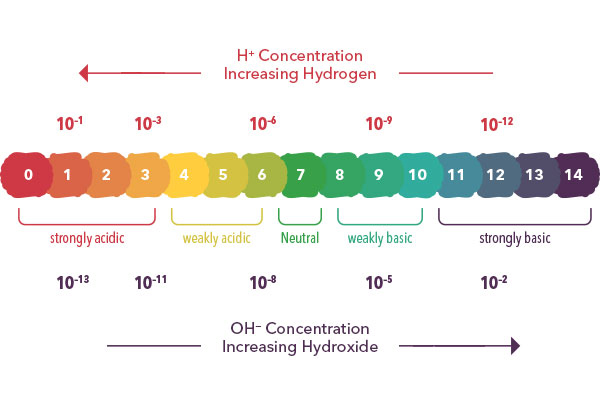The pH scale is logarithmic and as a result, each whole pH value below 7 is ten times more acidic than the next higher value. For example, pH 4 is ten times more acidic than pH 5 and 100 times (10 times 10) more acidic than pH 6. The same holds true for pH values above 7, each of which is ten times more alkaline (another way to say basic) than the next lower whole value. For example, pH 10 is ten times more alkaline than pH 9 and 100 times (10 times 10) more alkaline than pH 8.

# Theory of pH Electrodes

pH sensor measurements are made by comparing the readings in a sample with the readings in standards whose pH has been defined, frequently pH 4, 7, and 10 buffers. When the electrode is submerged in a sample, a potential develops across the sensing membrane surface and that membrane potential varies with differing pH values. A reference electrode provides a second, unvarying potential to quantitatively compare the changes of the sensing membrane potential. Combination pH electrodes are composed of a sensing electrode with the reference electrode simply built into the same electrode housing. Combo electrodes provide the same selectivity and response as a half-cell system, but offer the convenience of working and maintaining only one electrode. A meter serves to show the reading and also calculates the difference between the reference and sensing electrode potentials in mV (millivolts). The mV's are then converted to pH units and shown on the display.

The behavior of the electrode is described by the Nernst equation, named after German physical chemist Walther Nernst. The Nernst equation gives a formula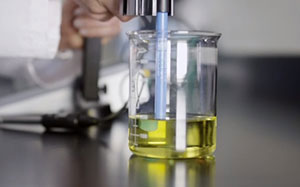that relates the numerical values of the concentration gradient to the electric gradient that balances it. For example, if a concentration gradient was established by dissolving KCl in half of a divided vessel that was originally full of H2O, and then a membrane permeable to K+ ions was introduced between the two halves—empirically, an equilibrium situation would arise where the chemical concentration gradient (that would normally cause ions to move from the region of high concentration to the region of low concentration) could be balanced by an electrical gradient that opposes the movement of charge.

E=Eo + (2.3 RT/nF) log aH+

E is the measured potential from the sensing electrode, Eo is related to the potential of the reference electrode, (2.3 RT/nF) is the Nernst factor and log aH+ is the pH. The Nernst factor, 2.3 RT/nF, includes the Gas Law constant (R), Faraday's constant (F), the temperature in degrees Kelvin (T) and the charge of the ion (n). For pH, where n=1, the Nernst factor is 2.3 RT/F, since R and F are constants, the factor and thusly the electrode behavior is dependent on temperature.

The electrode slope is a measure of the electrode response to the ion being detected and is equivalent to the Nernst factor. When the temperature is 25°C, the theoretical Nernst slope is 59.16 mV/pH unit. pH meters detect the pH sensing signal, reference signal, and temperature signal and then uses these values to calculate a pH value using the Nernst equation. Common buffers are typically used to calibrate the meter with known pH versus temperature values. This allows the meter to recognize a certain pH buffer and calibrate the slope and offset.

# Theory of pH Versus Temperature

The most common cause of error in pH measurements is temperature (assuming a clean electrode and fresh pH buffers are used). Temperature variations can influence pH for the following reasons:

The electrode slope will change with variations in temperature

Buffer and sample pH values will change with temperature

Measurement drift can occur when the internal elements of the pH and reference electrodes are reaching thermal equilibrium after a temperature change

When the pH electrode and temperature probe are placed into a sample that varies significantly in temperature, the measurements can drift because the temperature response of the pH electrode and temperature probe may not be similar if the sample does not have a uniform temperature, therefore, the pH electrode and temperature probe are responding differently

Electrode slope changes can be compensated for by using an automatic temperature compensation probe commonly referred to as ATC. pH meters calculate the electrode slope based on the measured temperature of the pH buffers. The meter will then automatically adjust the pH buffer value to the actual pH of the buffer at the measured temperature.

The pH values of the buffers and samples will change with variations in temperature because of their temperature dependent chemical equilibria. The pH electrode should be calibrated with buffers that have known pH values at different temperatures.

Nominal pH Value at 25C 0C 5C 10C 20C 30C 40C 50C 60C 70C 80C 90C
1.68 1.67 1.67 1.67 1.67 1.68 1.69 1.71 1.72 1.74 1.77 1.79
4.01 4.00 4.00 4.00 4.00 4.02 4.04 4.06 4.09 4.13 4.17 4.21
6.86 6.98 6.95 6.92 6.87 6.85 6.84 6.83 6.84 6.85 6.86 6.88
7.00 7.11 7.08 7.06 7.01 6.98 6.97 6.97 6.97 6.99 7.03 7.08
9.18 9.46 9.40 9.33 9.23 9.14 9.07 9.01 8.96 8.92 8.89 8.85
10.01 10.32 10.25 10.18 10.06 9.97 9.89 9.83 9.79 9.78 9.78 9.80
12.46 12.79 12.73 12.67 12.52 12.36 12.17 11.96 11.73 11.47 11.19 10.89

Several YSI instruments will measure pH in the laboratory, feel free to learn more about the new lab-specific pH meters in the TruLab and MultiLab family of products.# Additional Blog Posts of Interest:

Why is the pH Scale Logarithmic?

pH Meter Calibration Problems? Check Out These 12 Tips!

Extend the Life of Your pH Electrode in 3 Practical Steps

Laboratory Instruments and Electrodes for pH, ORP and ISEs with YSI TruLab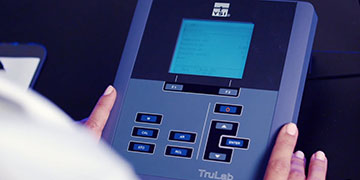Lab Instruments and Electrodes for pH, ORP and ISEs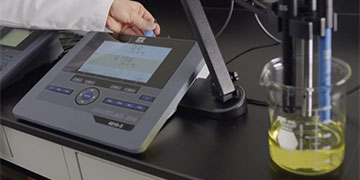pH Electrode Reference Systems - A Brief Primer Why You Need It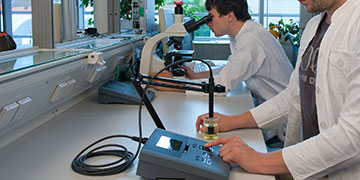4 Steps to GLP Compliant pH Measurement and Performance

## 0 Responses to this article

#### Add a comment

Your comment will appear after it is reviewed.

By clicking on Submit you agree that Xylem may use your personal data to aid in providing you support, and may contact you directly on this matter. Please have a look at our Privacy and Cookie Policy for more information on how/why and where we use your data